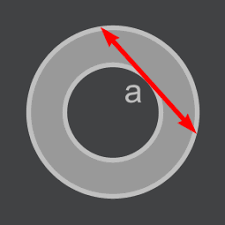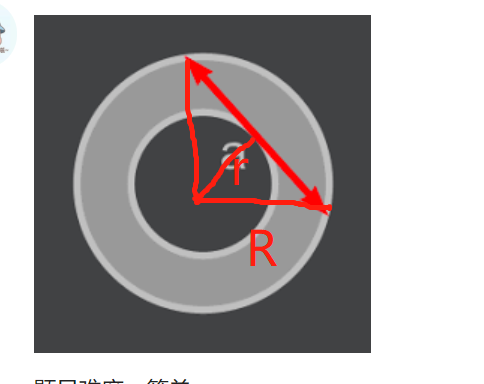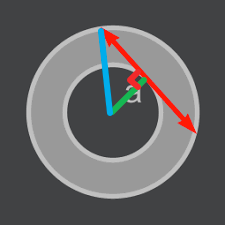【每日一题1203】环形面积

1. 线段长度a 是大于1的整数。
2. 圆周率请使用内置常量，例如Python中使用math.pidef solution(a: int) -> float:

assert solution(7) == 38.48
assert solution(13) == 132.73
import math

def solution(a: int) -> float:
area = (a / 2) ** 2 * math.pi
return round(area, 2)

assert solution(7) == 38.48
assert solution(13) == 132.73
import math
# 圆环面积 = PI * R ** 2 - PI * r ** 2
#                 = (R ** 2 - r**2) * PI
# 根据勾股定理得  : a / 2 ** 2 * PI
def solution(a: int) -> float:

return float('%.2f' % ((a / 2) ** 2 * math.pi))

assert solution(7) == 38.48
assert solution(13) == 132.73def solution(a: int) -> float:
return round((a / 2) ** 2 * math.pi, 2)

assert solution(7) == 38.48
assert solution(13) == 132.73

def solution(a: int) → float:
i = float(format((1/4)aa*pi, ‘.2f’))
return i

def solution(a: int) -> float:
# y*y + a/2 * a/2 = x * x
#wai = math.pi * (y*y + a/2 * a/2)
#nei = math.pi * y*y
return round(math.pi * a/2 * a/2,2)

Python 参考题解：

def solution(a: int) -> float:
import math

return round(math.pi * (a / 2) ** 2, 2)

assert solution(7) == 38.48
assert solution(13) == 132.73import math
from decimal import Decimal

def solution(a: int) -> float:
result = math.pi * (a / 2) ** 2
return float(Decimal(result).quantize(Decimal("0.00")))

assert solution(7) == 38.48
assert solution(13) == 132.73